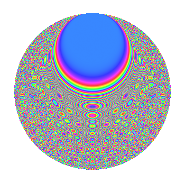# Properties

 Label 1008.2.dmLevel 1008 Weight 2 Character orbit dm Rep. character $$\chi_{1008}(155,\cdot)$$ Character field $$\Q(\zeta_{12})$$ Dimension 576 Sturm bound 384

# Related objects

## Defining parameters

 Level: $$N$$ = $$1008 = 2^{4} \cdot 3^{2} \cdot 7$$ Weight: $$k$$ = $$2$$ Character orbit: $$[\chi]$$ = 1008.dm (of order $$12$$ and degree $$4$$) Character conductor: $$\operatorname{cond}(\chi)$$ = $$144$$ Character field: $$\Q(\zeta_{12})$$ Sturm bound: $$384$$

## Dimensions

The following table gives the dimensions of various subspaces of $$M_{2}(1008, [\chi])$$.

Total New Old
Modular forms 784 576 208
Cusp forms 752 576 176
Eisenstein series 32 0 32

## Trace form

 $$576q - 12q^{6} + O(q^{10})$$ $$576q - 12q^{6} + 24q^{12} + 84q^{20} + 32q^{24} + 24q^{27} + 48q^{30} + 8q^{36} + 48q^{39} + 48q^{46} - 52q^{48} - 288q^{49} - 156q^{50} - 36q^{58} + 72q^{59} - 68q^{60} + 72q^{64} - 184q^{66} - 156q^{68} + 4q^{72} - 84q^{74} + 112q^{75} + 24q^{76} - 116q^{78} + 72q^{82} - 60q^{86} - 112q^{87} - 48q^{88} + 8q^{90} - 228q^{92} + 48q^{93} + 32q^{96} - 64q^{99} + O(q^{100})$$

## Decomposition of $$S_{2}^{\mathrm{new}}(1008, [\chi])$$ into newform subspaces

The newforms in this space have not yet been added to the LMFDB.

## Decomposition of $$S_{2}^{\mathrm{old}}(1008, [\chi])$$ into lower level spaces

$$S_{2}^{\mathrm{old}}(1008, [\chi]) \cong$$ $$S_{2}^{\mathrm{new}}(144, [\chi])$$$$^{\oplus 2}$$

## Hecke Characteristic Polynomials

There are no characteristic polynomials of Hecke operators in the database